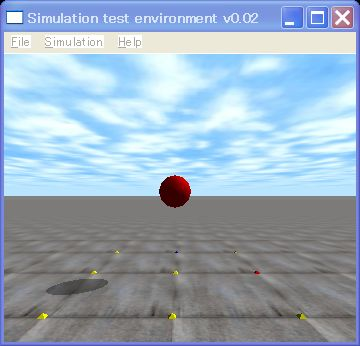# ODE Tutorial 5: Hello a Simulation World !

This time,  I show a super simple sample program which uses ODE (Open Dynamics Engine) .  In many programming books, printing “Hellow World” is the first sample program.  For physics simulation programming books, I think a simulation of free fall is the most simple  example.A screen shot of  free fall

[code]
// hello.cpp by Kosei Demura

#ifdef dDOUBLE
#define dsDrawSphere dsDrawSphereD
#endif

static dWorldID world; // For kinetics calculation
dBodyID ball; // Ball
const dReal radius = 0.2, mass = 1.0; // Radius of ball [m], weight of ball [kg]

// Simulation loop is called and executed each simlation loop.
// dynamics is calculated by dWorldStep().
static void simLoop (int pause)
{
const dReal *pos, *R;

// If you want more accuracy, you should set step size more small
// in this case, step size is 0.01 [s]
dWorldStep (world,0.01);

dsSetColor (1.0,0.0,0.0); // Setting of red (R, G, B)
pos = dBodyGetPosition (ball); // Getting the position of the ball
R = dBodyGetRotation (ball); // Getting the orientation of the ball
dsDrawSphere (pos, R, radius); // Drawing the sphere as the ball
}

void START ()
{ //setting of camera
static float xyz [ 3 ] = {0.0, -3.0,1.0}; // Position of a view point [m]
static float hpr [ 3 ] = {90.0,0.0,0.0}; // Direction of a gaze [°]
dsSetViewpoint (xyz, hpr); // Setting of the view point and the gaze
}

int main (int argc, char **argv)
{
dReal x0 = 0.0, y0 = 0.0, dReal z0 = 1.0; // Initial position of a ball
dMass m1;

dsFunctions fn; // Class for draw staff
fn.version = DS_VERSION; // Version of draw staff
fn.start = &start; // Preprocessing function of simulation
fn.step = &simLoop; // A function is called with each step of simulation
fn.command = NULL; // A function for a key board
// In the case of no function, appointing the NULL pointer
fn.stop = NULL; //
fn.path_to_textures = “../.. /drawstuff/textures”; // Path to the texture files

// Creation of the world for dynamics calculation
world = dWorldCreate ();
dWorldSetGravity (world,0,0, -0.001); // Acceleration of free fall [m/s^2]

// Creation of a ball
body = dBodyCreate (world); // Creation of a ball
dMassSetZero (&m1); // Initialization of a weight parameter m1
dMassSetSphereTotal (&m1, mass, radius); // Calculating m1 of the ball
dBodySetMass (ball, &m1); // Setting m1 to the ball
dBodySetPosition (ball, x0, y0, z0); // Setting the position of the ball

// Simulation loop
dsSimulationLoop (argc, argv,352,288, &fn); // Window Size 352 x 288

// Destruction of the world
dWorldDestroy (world);

return 0;
}
[/code]

This is the program of free fall of the red ball.  “world” is the software container where kinetics is calculated. Being for the first time to experient the world of physics, let’s call this sample program as “Hello World”.

The function which starts with the small letter ‘d’  is the API (Application Program Interface), and the function which starts with ‘ds’ is the API of  drawstuff. Drawstuff is the library for ODE, to test sample programs.

Execution method
2. From now on please execute the command below with the terminal. The \$ is prompt.
cd /home/ user name /src/ode/ode
tar xvzf sample1.tgz
cd  sample1
make
3. Execution
\$. /sample1

The red ball is falling slowly, but passing through the ground, and vanished.
To tell the truth it meaning that collision detection function is not installed in the program above.

The next time, collision detection function is added in this program.

english
スポンサーリンク
demura.netをフォローするdemura.net

## コメント

1. demu より:

Hello Anonymous,

I wrote some articles how to compile with ODE 0.10 in Japanese. Please see or translate by a machine.
http://demura.net/wordpress/tutorials/ode2

I know I must change this article, but it takes some time.

Cheers,

demu

2. 匿名 より:

hello
i dont know whats wrong with the make file but i get

c:\mingw\bin\..\lib\gcc\mingw32\3.4.5\..\..\..\..\mingw32\bin\ld.exe: cannot find -ldrawstuff

im using last version of ode..

3. demu より:

Hi Chengsen !

Time step 0.1 is too big.
Please set more small value, it improves accuracy.

demu

4. Chengsen より:

Dear demu,
May I ask a question on ODE here?
I want to simulate a sphere falling down from position (0,0,0), without initial velocity. The sphere is on earth (g = 9.8m/s/s), and the simulation step is 0.1s.

(1) Here is my program.

#include

#include

#define time_step (float)0.1

int main()

{

dWorldID myWorld_id;

dBodyID mySphere_id;

dMass sphereMass;

const dReal *pos;

float time = 0.0;

myWorld_id = dWorldCreate();

mySphere_id = dBodyCreate( myWorld_id );

dWorldSetGravity( myWorld_id, 0, 0, -9.8 );

dBodySetPosition( mySphere_id, 0, 0, 0 );

dMassSetSphere( &sphereMass, 1, 2 );

dBodySetMass( mySphere_id, &sphereMass );

dBodySetLinearVel( mySphere_id, 0.0, 0.0, 0.0 );

while (time < 1.0) {

static int i = 0;

dWorldStep( myWorld_id, time_step );

pos = dBodyGetPosition( mySphere_id );

std::cout <<i++ << ” :position (” << pos << “, ”

<< pos << “, ” << pos << “)\n”;

time += time_step;

}

dBodyDestroy( mySphere_id );

dWorldDestroy( myWorld_id );

return 0;

}

(2) The output is,

0 :position (0, 0, -0.098)

1 :position (0, 0, -0.294)

2 :position (0, 0, -0.588)

3 :position (0, 0, -0.98)

4 :position (0, 0, -1.47)

5 :position (0, 0, -2.058)

6 :position (0, 0, -2.744)

7 :position (0, 0, -3.528)

8 :position (0, 0, -4.41)

9 :position (0, 0, -5.39)

(3) My question is, why the z position of the sphere is not equal to h=1/2*g*t*t, which will be -0.049, -0.196,……?

Would you please kindly tell me the meaning of the output?

Chengsen

5. demu より:

Thank you very much for buying my book.

Now, I stay in Seoul to demonstrate my RoboCup humanoid soccer robot.
I will demonstrate in Indiana Hall, COEX, Seoul at 13:30 and 14:30 in 21 Octorber, 2007.

demu

6. Kime jeong soo より:

Hello

My name is jeong soo kim in korea.

I want to learn ODE.